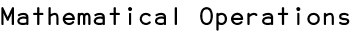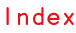RSS

Scriptographer allows you to write normal algebraic operators in connection with basic type objects. Points and Sizes can be added to, subtracted from, multiplied with or divided by numeric values or other points and sizes:

```// Define a point to start with
var point1 = new Point(10, 20);

// Create a second point that is 4 times the first one.
// This is the same as creating a new point with x and y
// of point1 multiplied by 4:
var point2 = point1 * 4;
console.log(point2); // { x: 40, y: 80 }

// Now we calculate the difference between the two.
var point3 = point2 - point1;
console.log(point3); // { x: 30, y: 60 }

// Create yet another point, with a numeric value added to point3:
var point4 = point3 + 30;
console.log(point4); // { x: 60, y: 90 }

// How about a third of that?
var point5 = point4 / 3;
console.log(point5); // { x: 20, y: 30 }

// Multiplying two points with each other multiplies each
// coordinate seperately
var point6 = point5 * new Point(3, 2);
console.log(point6); // { x: 60, y: 60 }
```

These operators work just fine in conjunction with the feature of object conversion as described in the Object Conversion tutorial, as long as the object the operation is performed on is a real basic type:

```var point1 = new Point(10, 20);
var point2 = point1 + { x: 100, y: 100 };
console.log(point2); // { x: 110, y: 120 }

// Adding size objects to points work too,
// forcing them to be converted to a point first
var point3 = point2 + new Size(50, 100);
console.log(point3); // { x: 160, y: 220 }

// And using the object notation for size works just as well:
var point4 = point3 + { width: 40, height: 80 };
console.log(point4); // { x: 200, y: 300 }

var point5 = point4 + [100, 0];
console.log(point5); // { x: 300, y: 300 }
```

## Math Functions

Scriptographer offers several mathematical functions for rounding the values of points and sizes:

```var point = new Point(1.2, 1.8);

// Round the point:
var rounded = point.round();
console.log(rounded); // { x: 1, y: 2 }

// Round the point up:
var ceiled = point.ceil();
console.log(ceiled); // { x: 2, y: 2 }

// Round the point down:
var floored = point.floor();
console.log(floored); // { x: 1, y: 1 }
```

Use Point.random() or Size.random() to create a point or size with random values for each of its properties between 0 and 1:

```// Create a point whose x is between 0 and 50,
// and y is between 0 and 100
var point = new Point(50, 100) * Point.random();

// Create a size whose width is between 0 and 50,
// and height is between 0 and 100
var size = new Size(50, 100) * Size.random();
```